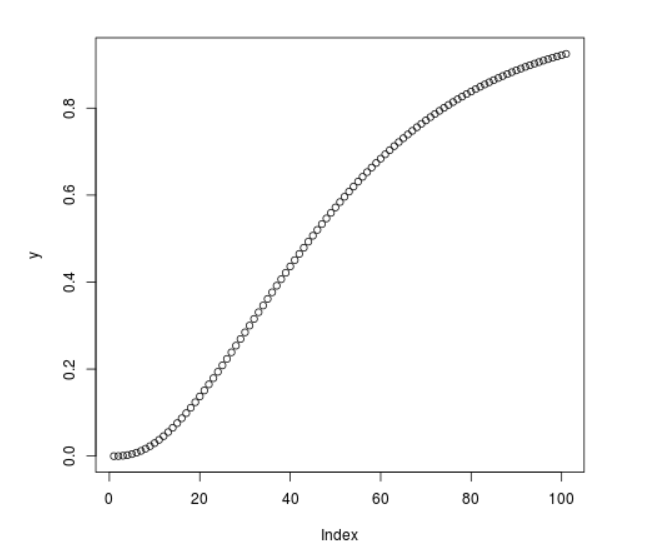Related Articles
Compute Cumulative Chi Square Density in R Programming – pchisq() Function
• Last Updated : 25 Jun, 2020

`pchisq()` function in R Language is used to compute cumulative chi square density for a vector of elements. It also creates a density plot for chi square cumulative distribution.

Syntax: pchisq(vec, df)

Parameters:
vec: Vector of x-values
df: Degree of Freedom

Example 1:

 `# R program to compute  ` `# Cumulative Chi Square Density ` ` `  `# Create a vector of x-values ` `x <``-` `seq(``0``, ``10``, by ``=` `1``) ` ` `  `# Calling pchisq() Function ` `y <``-` `pchisq(x, df ``=` `5``) ` `y `

Output:

```  0.00000000 0.03743423 0.15085496 0.30001416 0.45058405 0.58411981
 0.69378108 0.77935969 0.84376437 0.89093584 0.92476475
```

Example 2:

 `# R program to compute  ` `# Cumulative Chi Square Density ` ` `  `# Create a vector of x-values ` `x <``-` `seq(``0``, ``10``, by ``=` `0.1``) ` ` `  `# Calling pchisq() Function ` `y <``-` `pchisq(x, df ``=` `5``) ` ` `  `# Plot a graph  ` `plot(y) `

Output:My Personal Notes arrow_drop_up
Recommended Articles
Page :